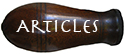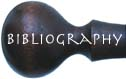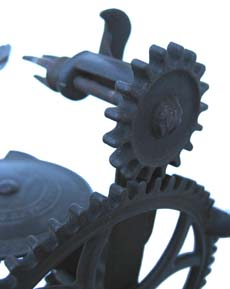# Gear RatiosIn this inverted Reading (left) a larger hand-driven gear meshes with a smaller gear turning the fork, which provides a speed advantage. With one turn of the hand-driven gear, the fork and apple rotate many times. One can determine just how many times by calculating the gear ratio. The gear ratio can be determined by counting the teeth on the gears. In this case, the hand-driven gear has 55 teeth and the fork gear has 17. Divide 55 by 17 and you can see the apple will rotate 3.2 times for every complete turn of the hand-driven gear.In this same parer a steel axle connects the hand-driven gear to a smaller table gear (pictured to the right). Even though the two gears are of different size they rotate at the same rate because they are on the same axle. The smaller table gear rotates a larger turntable gear, which provides a mechanical advantage. We can determine how many times the hand-driven gear/shaft/table gear assembly must be turned to rotate the turntable one time by calculating the gear ratio. The turntable has 45 teeth and the small table gear rotating it has 9 teeth. Divide 45 by 9 and we can see the small table gear and hence the hand-driven gear must make 5 complete turns to rotate the turntable once. So, roughly half of these 5 turns will be used to pare the apple and the remaining turns used to place the paring blade back into starting position.

The axle of the small table gear is at a 90-degree angle from the axle of the larger turntable gear. This gear arrangement is used to change the direction of motion.